# KCET 2015 Physics Paper with Solutions

KCET 2015 Physics question paper with solutions are available on this page. The questions have been solved our experts and they have come up with appropriate solutions for each of the question asked in the 2015 Physics paper. Students can have a look at them and at the same time practise solving these questions to get familiar with the question paper pattern, difficulty level of the questions, understand the important topics. Students will also develop better speed and accuracy for solving the problems.The KCET 2015 Physics question paper along with the solutions are available in a PDF format and download option is present at the bottom of this page.

### KCET 2015 - Physics

Question 1: The ratio of the dimensions of Planck constant and that of moment of inertia has the dimensions of

1. a. time
2. b. frequency
3. c. angular momentum
4. d. velocity

Solution:

E = h ν

$$h = \frac{E}{\upsilon }=\frac{\left [ ML^{2}T^{-2} \right ]}{\left [ T^{-1} \right ]}=ML^{2}T^{-1}$$
-----------(1)

Moment of Inertia I = mr2 = [ML2] ----------(2)

Dividing eq. (1) and (2)

$$\left [ \frac{h}{I} \right ]=\frac{\left [ ML^{2}T^{-1} \right ]}{\left [ML^{2} \right ]}=\left [ T^{-1} \right ]$$

[T-1] = frequency of a particle.

Question 2: The velocity-time graph for two bodies A and B are shown. Then the acceleration of A and B are in the ratio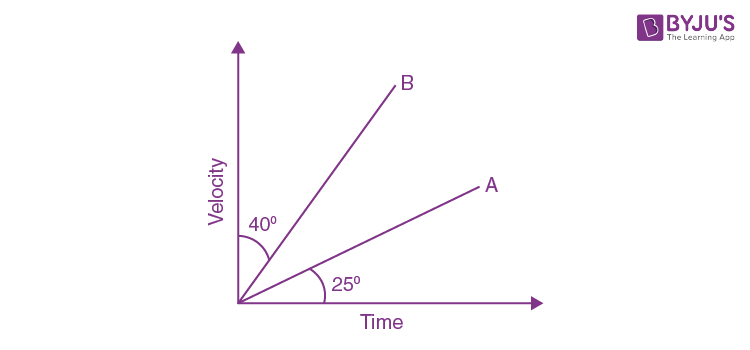1. a. tan25° to tan 40°
2. b. tan 25° to tan 50°
3. c. sin 25° to sin 50°
4. d. cos 25° to cos 50°

Solution:

Acceleration, a = ΔV/Δt

So, acceleration of body A = aA = tan25°

Line B makes an angle of 50°. So its slope is equal to tan 50°.

aB = tan 50°

aA :aB = tan 25° : tan 50°

Question 3: A particle is projected with a velocity v so that its horizontal range is twice the greatest height attained. The horizontal range is

1. a. v2/g
2. b. 2v2/3g
3. c. 4v2/5g
4. d. v2/2g

Solution: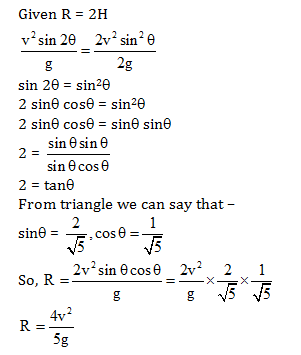Question 4: A stone of mass 0.05 kg is thrown vertically upwards. What is the direction and magnitude of net force on the stone during its upward motion?

1. a. 0.49 N vertically upwards
2. b. 0.49 N vertically downwards
3. c. 0.98 N vertically downwards
4. d. 9.8 N vertically downwards

Solution:

F = mg

Here m = 0.05 kg

F = 0.05 × (–9.8) = – 0.49N

F = 0.49 N vertically downwards

Question 5: The kinetic energy of a body of mass 4 kg and momentum 6 Ns will be

1. a. 2.5 J
2. b. 3.5 J
3. c. 4.5 J
4. d. 5.5 J

Solution:

Given m = 4kg, p = 6 Ns

$$K.E = \frac{p^{2}}{2m}=\frac{6\times 6}{2\times 4}=\frac{0.36}{8}$$

K.E. = 4.5 J

Question 6: The ratio of angular speed of a second-hand to the hour-hand of a watch is

1. a. 720:1
2. b. 60:1
3. c. 3600:1
4. d. 72:1

Solution:

Angular velocity = 2λ/60 = λ/30

For an hour hand to complete 1 rotation i.e. 2π radians it takes 12 hrs.

So angular velocity of an hour hand = 2λ/(12 x 3600)

= λ/(21600)

Then the ratio is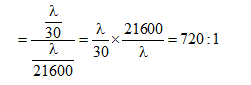Question 7: If the mass of a body is M on the surface of the earth, the mass of the same body on the surface of the moon will be

1. a. M/6
2. b. M
3. c. 6 M
4. d. zero

Solution:

As we know that the value of acceleration due to gravity is independent of mass, shape, size etc. of the body and depends upon the mass and radius of the earth.

Question 8: Moment of Inertia of a thin uniform rod rotating about the perpendicular axis passing through its centre is 1. If the same rod is bent into a ring and its moment of inertia about its diameter is F. Then the ratio I/I’ will be

1. a. (3/2) π2
2. b. (8/3) π2
3. c. (2/3) π2
4. d. (5/3) π2

Solution: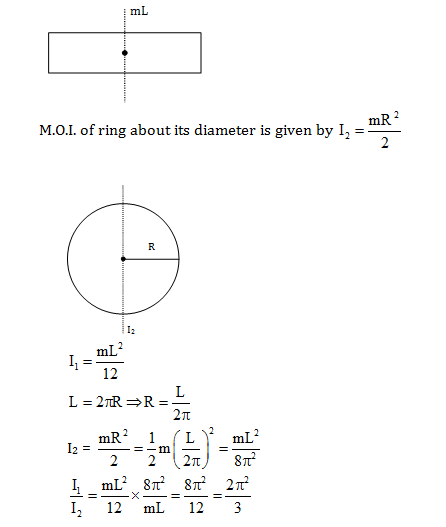Question 9: The ratio of hydraulic stress to the corresponding strain is known as

1. a. Compressibility
2. b. Bulk modulus
3. c. Young's modulus
4. d. Rigidity modulus

Solution:

The ratio of hydraulic stress to the corresponding strain is known so bulk modulus.

Bulk modulus [K] = hydraulic stress/strain

Question 10: The efficiency of a Carnot engine which operates between the two temperatures T1 = 500 K and T2 = 300 K is

1. a.50%
2. b.25%
3. c.75%
4. d.40%

Solution:

T1 = 500K, T2 = 300K

Efficiency of Carnot engine, η = 1 – (T2/T1)

$$\eta =1-\frac{300}{500}=\frac{500-300}{500}= \frac{200}{500}$$

η = (2/5) =0.4

So, the efficiency is 40%.

Question 11: Water is heated from 0 °C to 10 °C then its volume

1. a. decreases
2. b. increases
3. c. does not change
4. d. first decreases and then increases

Solution:

When water is heated from 0°C to 4°C, there is a decreasing amount in volume and density increases till it is a 4°C and then volume starts increasing to 10°C.

Question 12: 1 gram of ice is mixed with 1 gram of steam. At thermal equilibrium, the temperature of the mixture is

1. a. 0 °C
2. b. 100 °C
3. c. 50 °C
4. d. 55 °C

Solution:

(1) Ice convert into water

Q1 = M1Lf = 1 × 80 = 80 cal

(2) Steam to convert into water

Q2 = M2Lv = 1 × 540 = 540 cal

(3) 1g of water at 0°C into water at 100°C

=1 × 1 × 100 = 100 cal

So clearly, the whole steam is not condensed. So the temperature of the mixture is 100°C.

Question 13: The ratio of kinetic energy to the potential energy of a particle executing SHM at a distance is equal to half of its amplitude, the distance being measured from its equilibrium position will be

1. a. 3 : 1
2. b. 4 : 1
3. c. 2 : 1
4. d. 8 : 1

Solution: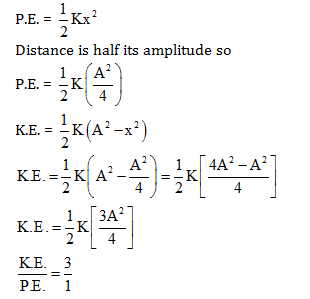Question 14: When two tuning forks A and B are sounded together. 4 beats per second are heard. The frequency of fork B is 384 Hz. When one of the prongs of the fork A is filed and sounded with B. the beat frequency increases, then the frequency of the fork A is

1. a. 380 Hz
2. b. 378 Hz
3. c. 379 Hz
4. d. 389 Hz

Solution:

Beat frequency

$$b=\left | \nu _{A}- \nu _{B}\right |$$

4 = υA – 384

υA = 388 Hz

Question 15: A stretched string is vibrating in the second overtone, then the number of nodes and antinodes between the ends of the string are respectively

1. a. 4 and 3
2. b. 3 and 2
3. c. 3 and 4
4. d. 2 and 3

Solution:

From the figure, number of nodes and antinodes will be 4 and 3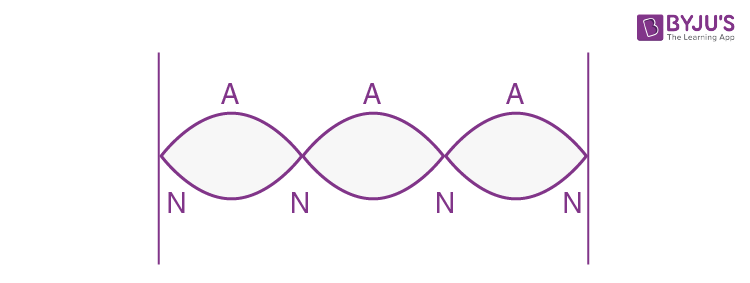Question 16: Two spheres carrying charges + 6 μC and + 9 μC separated by a distance d, experiences a force of repulsion F. When a charge of –3 μC is given to both the sphere and kept at the same distance as before, the new force of repulsion is

1. a. F
2. b. 3F
3. c. F/3
4. d. F/9

Solution:

$$F_{1}=\frac{Kq_{1}q_{2}}{r^{2}}=\frac{K(9)(6)}{d^{2}}$$

$$\Rightarrow \frac{K}{d^{2}}=\frac{F_{1}}{54}$$

Now charges are 3μC and 6μC

So, new force is

$$F_{2}= \frac{K(6)(3)}{d^{2}}=\frac{18K}{d^{2}}$$

Put the value of K/d2 in F2

$$F_{2}= 18 \times \frac{F_{1}}{54}=\frac{F}{3}$$

Question 17: Pick out the statement which is incorrect.

1. a. The tangent drawn to a line of force represents the direction of the electric field
2. b. The electric field lines form a closed loop
3. c. A negative test charge experiences a force opposite to the direction of the field
4. d. Field lines never intersect

Solution:

Electric field lines do not form a closed loop as the line can never start and end on the same change.

Question 18: The angle between the dipole moment and electric field at any point on the equatorial plane is

1. a. 0°
2. b. 90°
3. c. 180°
4. d. 45°

Solution:

The angle between electric dipole moment and electric field in the equatorial line is 180° as both of them are in opposite directions.

Question 19: Three point charges 3nC, 6nC and 9nC are placed at the corners of an equilateral triangle of side 0.1 in. The potential energy of the system is

1. a. 8910 J
2. b. 89100 J
3. c. 9910 J
4. d. 99100 J

Solution:

Question 20: A spherical shell of radius 10 cm is carrying a charge q. If the electric potential at distances 5 cm, 10 cm and 15 cm from the centre of the spherical shell is V1, V2 and V3 respectively, then

1. a. V1> V2 > V3
2. b. V1< V2 < V3
3. c. V1 = V2 > V3
4. d. V1 = V2 < V3

Solution:

Inside the spherical shell, potential is same.

V1 = V2

V ∝ (1/d)

V1 = V2 > V3

Question 21: A parallel plate capacitor is charged and then isolated. The effect of increasing the plate separation on charge, potential and capacitance respectively are

1. a. constant, decreases, decreases
2. b. increases, decreases, decreases
3. c. constant, decreases, increases
4. d. constant, increases, decreases

Solution:

From

$$C = \frac{\varepsilon _{0}A}{d}$$

When it increases the plate separation capacitance decrease and by V = Q/C

V also increases and q is constant.

Question 22: Four identical cells of emf E and internal resistance r are to be connected in series. Suppose if one of the cells is connected wrongly. The equivalent emf and effective internal resistance of the combination is

1. a. 4E and 4r
2. b. 4E and 2r
3. c. 2E and 4r
4. d. 2E and 2r

Solution:

Resistance in series connection -

req = r1 + r2 + r3 + r4 = r + r + r + r = 4r

emf in series connection –

Eeq = E1 + E2 + E3 – E4 (When one is connected wrong)

Eeq = E + E + E – E = 2E

Question 23: Three resistances 2Ω, 3Ω and 4Ω are connected in parallel. The ratio of currents passing through them when a potential difference is applied across its ends will be

1. a. 6 : 3 : 2
2. b. 6 : 4 : 3
3. c. 5 : 4 : 3
4. d. 4 : 3 : 2

Solution:Current through 4 Ω = I1 = V/4

Current though 3 Ω = I2 = V/4

Similarly, I3 = V/2

$$I_{1}:I_{2}:I_{3}=\frac{V}{4}:\frac{V}{3}:\frac{V}{2}$$

$$I_{1}:I_{2}:I_{3}=\frac{1}{4}:\frac{1}{3}:\frac{1}{2}$$

I1:I2:I3 = 6:4:3

Question 24: Two cells of emf E1 and E2 are joined in opposition (such that E1> E2). If r1 and r2 be the internal resistances and R will be the external resistance, then the terminal potential difference is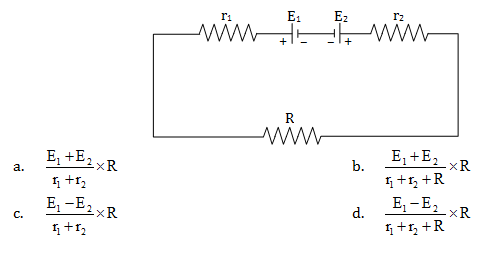Solution:

Two cells of emf E1 and E2 are joined in opposition E1> E2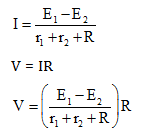Question 25: In the circuit shown below, the ammeter and the voltmeter readings are 3 A and 6 V respectively. Then the value of the resistance R is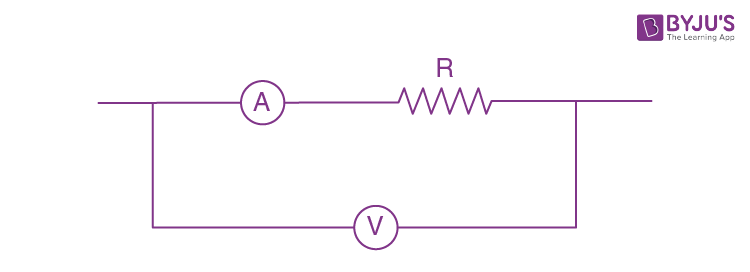1. a. 2 Ω
2. b. > 2 Ω
3. c. < 2 Ω
4. d. ≥ 2 Ω

Solution:

R = V/I = 6/3 = 2 Ω (For ideal ammeter and voltmeter)

R < 2 Ω when A and V are infinite

3(R + r) = 6V

R + r = 2

R = 2 – r

Question 26: In Wheatstone's network P = 2 Ω, Q = 2 Ω, R = 2 Ω and S = 3 Ω. The resistance with which S is to be shunted in order that the bridge may be balanced is

1. a. 1Ω
2. b. 2Ω
3. c. 4 Ω
4. d. 6 Ω

Solution: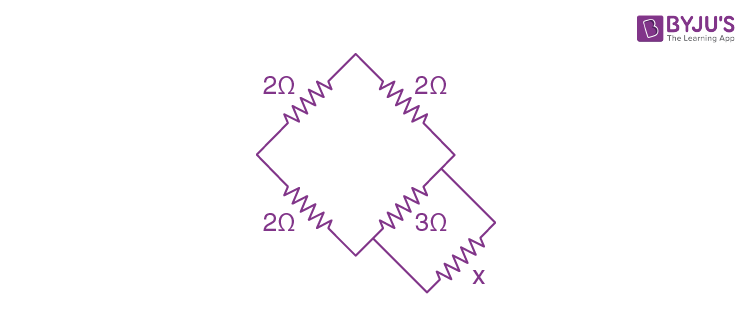$$\frac{2}{2}=\frac{2}{\frac{3x}{3+x}}$$

$$\Rightarrow 1=\frac{2}{1}\times \frac{3+x}{3x}$$

3x = 6 + 2x

x = 6 Ω

Question 27: The resistance of the bulb filament is 100 Ω at a temperature of 100 °C. If its temperature coefficient of resistance be 0.005 per °C, its resistance will become 200 Ω at a temperature

1. a. 300°C
2. b. 400°C
3. c. 500°C
4. d. 200°C

Solution:

R = R0 (1+ α ΔT)

200 = 100(1+0.005(T2-100))

2 = (1+0.005 T2 - 0.5)

2 = 0.5 + 0.005T2

T2 = 1.5/0.005

T2 = 300°C

Question 28: Two concentric coils each of radius equal to 2π cm are placed right angles to each other. If 3A and 4A are the currents flowing through the two coils respectively. The magnetic induction (in Wb m–2) at the centre of the coils will be

1. a. 12 × 10–5
2. b. 10–5
3. c. 5 × 10–5
4. d. 7 × 10–5

Solution: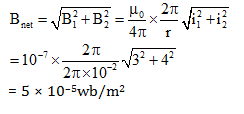Question 29: A proton beam enters a magnetic field of 10–4 Wb m–2 normally. if the specific charge of the proton is 1011 C kg–1 and its velocity is 109ms–1, then the radius of the circle described will be

1. a. 0.1 m
2. b. 10 m
3. c. 100 m
4. d. 1 m

Solution:

Given B = 10–4 Wb/m2

Q = 1011 C/kg, v = 109 m/sec

Radius of circle described, r = mV/qB

$$r=\frac{1\times 10^{9}}{10^{11}\times 10^{-4}}=\frac{10^{13}}{10^{11}}=10^{2}$$

r = 100 m

Question 30: A cyclotron is used to accelerate

1. a. neutron
2. b. only positively charged particles
3. c. only negatively charged particles
4. d. both positively and negatively charged particles

Solution:

A cyclotron is used to accelerate both positively and negatively charged particles but a neutral particle cannot be accelerated in a cyclotron.

Question 31: A galvanometer of resistance 50 Ω gives a full-scale deflection for a current 5 × 104 A. The resistance that should be connected in series with the galvanometer to read 3 V is

1. a. 595 Ω
2. b. 5050 Ω
3. c. 5059 Ω
4. d. 5950 Ω

Solution: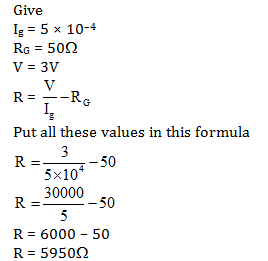Question 32: Two parallel wires 1 m apart carry currents of 1 A and 3 A respectively in opposite directions. The force per unit length acting between these two wires is

1. a. 6 × 10–7 Nm–1 repulsive
2. b. 6 × 10–7 Nm–1 attractive
3. c. 6 × 10–5 Nm–1 repulsive
4. d. 6 × 10–5 Nm–1 attractive

Solution:

I1 = 1A, I2 = 3A, d = 1m

$$F=\frac{\mu _{0}}{4x}\frac{2I_{1}I_{2}}{d}$$

$$F=10^{-7}\times \frac{2(1)(3)}{1}$$

F = 6 × 10–7 N/m

Current flowing in opposite direction is repulsive in nature.

Question 33: If there is no torsion in the suspension thread, then the time period of a magnet executing SHM is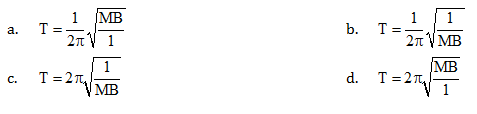Solution:

If there is no torsion in the suspension thread, then the time period of a magnet executing S.H.M. is

$$T=2\pi \sqrt{\frac{I}{MB}}$$

Question 34: Core of electromagnets are made of ferromagnetic material which has

1. a. high permeability and low retentivity
2. b. high permeability and high retentivity
3. c. low permeability and high retentivity
4. d. low permeability and low retentivity

Solution:

Electromagnetic cores support the formation of a magnetic field because of high permeability and low retentivity so that the magnetic field gets demagnetized easily.

Question 35: The magnetic susceptibility of a paramagnetic material at –73 °C is 0.0075 and its value at –173 °C will be

1. a. 0.0045
2. b. 0.0030
3. c. 0.015
4. d. 0.0075

Solution:

Given x1 = 0.0075

T1 = -730C = 273-73 = 200k

T2 = -1730C = 273-173 = 100k

X1T1 = X2 T2

(0.0075) (200) = X2 (100)

X2 = 0.015

Question 36: Two coils have a mutual inductance 0.005 H. The current changes in the first coil according to the equation i = im sin ωt where im= 10 A and ω = 100 π rad s–1. The maximum value of the emf induced in the second coil is

1. a. 2 π
2. b. 5 π
3. c. π
4. d. 4 π

Solution: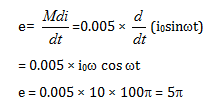Question 37: An aircraft with a wingspan of 40 m flies with a speed of 1080 km/hr in the eastward direction at a constant altitude in the northern hemisphere, where the vertical component of the earth's magnetic field is 1.75 × 10–5 T. Then the emf developed between the tips of the wings is

1. a. 0.5V
2. b. 0.34V
3. c. 0.21V
4. d. 2.1V

Solution:

Given B=1.75 ×10-5T.

l = 40m

V = 1080 km/h = 1080 ×(5/18) =300 m/sec

E=Bvl

E=(1.75×10-5)(300)(40)

E=(1.75×10-5)12000

E=2100×10-4

E= 0.21 volt

Question 38: In an LCR circuit, at resonance

1. a. the current and voltage are in phase
2. b. the impedance is maximum
3. c. the current is minimum
4. d. the current leads the voltage by π/2

Solution:

For resonance condition

XL = XC

In an L-C-R circuit at resonance, the current and voltage are in phase.

Question 39: A transformer is used to light 100 W –110 V lamp from 220 V mains. If the main current is 0.5 A, the efficiency of the transformer is

1. a. 90%
2. b. 95%
3. c. 96%
4. d. 99%

Solution:

P=VI

Efficiency of transformer, η = (P0/Pi) x 100

P0 = 100W

Pi = ViIi = 220 × 0.5 = 110W

η = (100/110) x 100 = 90.9%

So, the efficiency of the transformer is 90%

Question 40: The average power dissipated in a pure inductor is

1. a. (1/2) VI
2. b. VI2
3. c. VI2/4
4. d. 0

Solution:

Average power dissipated

$$P_{avg}=\frac{\nu _{0}i_{0}}{2}cos\phi$$

$$P_{avg}=\frac{\nu _{0}i_{0}}{2}cos90^{0}$$

Pavg =0

Question 41: If ε0 and μ0 are the permittivity and permeability of free space and ε and μ are the corresponding quantities for a medium, then refractive index of the medium is

1. a.
$$\sqrt{\frac{\mu _{0}\varepsilon _{0}}{\mu \varepsilon }}$$
2. b.
$$\sqrt\frac{\mu \varepsilon }{\mu _{0}\varepsilon _{0}}$$
3. c. 1
4. d. Insufficient information

Solution: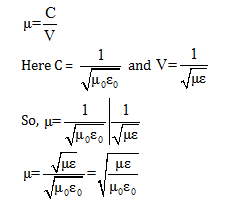Question 42: A person wants a real image of his own, 3 times enlarged. Where should he stand in front of a concave mirror of radius of curvature 30 cm?

1. a. 10 cm
2. b. 30 cm
3. c. 90 cm
4. d. 20 cm

Solution:

$$f=\frac{R}{2}=\frac{-30}{2}=-15cm$$

$$m=\frac{-\nu }{u}$$

$$\Rightarrow -3=\frac{-v }{u}$$

V = 3u

By mirror formula

$$-\frac{1}{F}=\frac{1}{v}+\frac{1}{u}$$

$$-\frac{1}{15}=\frac{1}{3u}+\frac{1}{u}=\frac{1+3}{3u}=\frac{4}{3u}$$

So,

$$\frac{4\times (-15)}{3}=-20cm$$

Question 43: Calculate the focal length of a reading glass of a person if his distance of distinct vision is 75 cm.

1. a. 25.6 cm
2. b. 37.5 cm
3. c. 75.2 cm
4. d. 100.4 cm

Solution:

Here V= –7.5 cm

u = –D = –25 cm

It is Because of distinct vision-

Using lens formula

$$\frac{1}{f}=\frac{1}{v}-\frac{1}{u}$$

$$\frac{1}{f}=\frac{1}{-75}+\frac{1}{25}=\frac{-1+3}{75}=\frac{2}{75}$$

f= (75/2) = 37.5 cm

Question 44: In Young's double-slit experiment, the slit separation is 0.5 m from the slits. For a monochromatic light of wavelength 500 nm, the distance of 3rd maxima from 2nd minima on the other side is

1. a. 2.75 mm
2. b. 2.5 mm
3. c. 22.5 mm
4. d. 2.25 mm

Solution:

Question 45: To observe diffraction, the size of the obstacle

1. a. has no relation to the wavelength
2. b. should be λ/2, where λ is the wavelength
3. c. should be much larger than the wavelength
4. d. should be of the order of the wavelength

Solution:

To observe diffraction the size of the obstacle should be of the order of wavelength because of λ

≈ d.

Question 46: The polarizing angle of glass is 57°. A ray of light which is incident at this angle will have an angle of refraction as

1. a. 25°
2. b. 33°
3. c. 43°
4. d. 38°

Solution:

Angle of incident i=57°

So, i = r = 57°

the reflected and refracted rays are mutually perpendicular to each other

So the angle of refraction r' = 90°–i

r' = 90°-57°=330

Question 47: Light of two different frequencies whose photons have energies 1 eV and 2.5 eV respectively, successively illuminate a metallic surface whose work function is 0.5 eV. Ratio of maximum speeds of emitted electrons will be

1. a. 1 : 5
2. b. 1 : 4
3. c. 1 : 2
4. d. 1 : 1

Solution: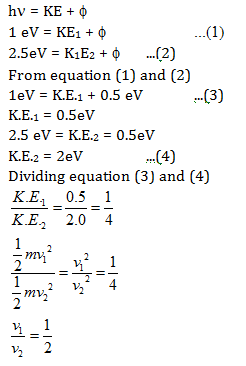Question 48: Find the de-Broglie wavelength of an electron with a kinetic energy of 120 eV.

1. a. 95 pm
2. b. 102 pm
3. c. 112 pm
4. d. 124 pm

Solution:

λ= h/p

Here

$$P = \sqrt{2M.K.E}=\sqrt{2\times 9\times 10^{-31}\times 120\times 1.6\times 10^{-19}}$$

P = 5.88 × 10-24 Kg MS-1

So, de Broglie wavelength

λ=h/p = (6.63 x 10-34/5.88 x 10-24)

= 1.13 × 10-10 m

= 1.13 Å

Question 49: An α-particle of energy 5 MeV is scattered through 180° by gold nucleus. The distance of closest approach is of the order of

1. a. 10–10cm
2. b. 10–12cm
3. c. 10–14cm
4. d.10–16cm

Solution:

$$5MeV = \frac{1}{4\pi \varepsilon _{0}}\frac{q_{1}q_{2}}{r}$$

$$5\times 10^{6}\times e = \frac{(9\times 10^{9})(92e)(2e)}{r}$$

$$5\times 10^{6}\times = \frac{(9\times 10^{9})(92)(2e)}{r}$$

$$r=\frac{(9\times 10^{9})(92)(2\times 1.6\times 10^{-19})}{5\times 10^{6}}$$

r = 5.3×10-14m = 5.3×10-12cm

Question 50: If an electron in a hydrogen atom jumps from an orbit of level n = 3 to an orbit of level n = 2, the emitted radiation has a frequency (R = Rydberg constant, C = velocity of light)

1. a. 3RC/27
2. b. RC/25
3. c. 8RC/9
4. d. 5RC/36

Solution: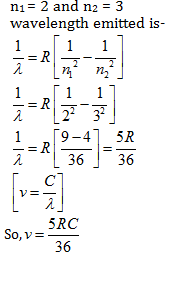Question 51: What is the wavelength of light for the least energetic photon emitted in the Lyman series of the hydrogen spectrum. (take he = 1240 eV nm)

1. a. 82 nm
2. b. 102 nm
3. c. 122 nm
4. d. 150 nm

Solution:

Given hc=1240 ev nm, R=1.09737×107 per meter

Wavelength of light for least – energetic photon emitted in Lyman series of hydrogen atom

$$\frac{1}{\lambda }=R\left ( \frac{1}{n_{2}^{2}}-\frac{1}{n_{1}^{2}} \right )$$

here n1 = 2 and n2 = 1, R = 0.01097 nm-1

$$\frac{1}{\lambda }=0.01097\left [ \frac{1}{1^{2}}-\frac{1}{2^{2}} \right ]$$

$$\frac{1}{\lambda }=0.01097\left [ \frac{4-1}{4}\right ]$$

$$\frac{1}{\lambda }=0.01097\left [ \frac{4-1}{4}\right ]$$

$$\lambda = \frac{4}{0.01097 \times 3}$$

λ = 121.5436

λ= 122nm

Question 52: A nucleus at rest splits into two nuclear parts having radii in the ratio 1 : 2.Their velocities are in the ratio

1. a. 8: 1
2. b. 6: 1
3. c. 4: 1
4. d. 2: I

Solution:

By conservation of linear momentum.

m1v1 = m2v2

$$\frac{v_{1}}{v_{2}}=\frac{m_{2}}{m_{1}}=\left ( \frac{R_{2}}{R_{1}} \right )^{3}$$

$$\frac{v_{1}}{v_{2}}=\left ( \frac{2}{1} \right )^{3}=8:1$$

Question 53: The half-life of a radioactive substance is 20 minutes. The time taken between 50 % decay and 87.5 % decay of the substance will be

1. a. 30 minutes
2. b. 40 minutes
3. c. 25 minutes
4. d. 10 minutes

Solution:

T1/2 = 20min

N1 = 50, N2 = 100-87.5=12.5

$$\Delta \lambda -\frac{T}{ln_{2}}ln\left [ \frac{N_{1}}{N_{2}} \right ]=\frac{20}{ln_{2}}ln\left [ \frac{50}{12.5} \right ]$$

n= 40 min

Question 54: A radioactive decay can form an isotope of the original nucleus with the emission of particles

1. a. one α and four β
2. b. one α and two β
3. c. one α and one β
4. d. four α and one β

Solution: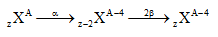Question 55: An LED is constructed from a pn junction based on a certain semi-conducting material whose energy gap is 1.9 eV. Then the wavelength of the emitted light is

1. a. 2.9 × 10–9 m
2. b. 1.6 × 10–8 m
3. c. 6.5 × 10–7 m
4. d. 9.1 × 10–5 m

Solution:

Eg = 1.9 eV, hc = 1240 eV

Wavelength = λ = (hc/Eg)nm

λ = (1240/1.9) = 652.63 ≈ 650 nm

= 6.5 × 10–7m

Question 56: Amplitude modulation has

1. a. one carrier with two sideband frequencies
2. b. one carrier
3. c. one carrier with infinite frequencies
4. d. one carrier with high frequency

Solution:

In amplitude modulation modulated signal has a carrier wave with two sideband frequencies one is lower sideband and other is upper side bad.

Question 57: The circuit has two oppositely connected ideal diodes in parallel. What is the current flowing in the circuit?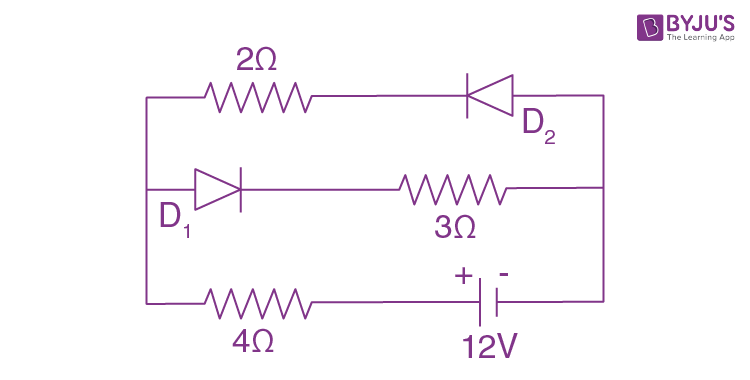1. a. 1.71 A
2. b. 2.0 A
3. c. 2.31 A
4. d. 1.33 A

Solution:

Diode D1 is forward biased but D2 is reversed biased. So no current flows through D2 but current flows through D1.

Req = 3 + 4 = 7 Ω

I = (V/ Req) = 12/7=1.71

Question 58: The input characteristics of a transistor in CE mode is the graph obtained by plotting

1. a. IB against VBE at constant VCE
2. b. IB against VCE at constant VBE
3. c. IB against IC at constant VCE
4. d. IB against IC at constant VBE

Solution:

Input characteristics circle is drawn between IB and VEB at constant VCE.

$$R =\left [\frac{\Delta V_{BE}}{\Delta I_{B}} \right ]_{V_{CE}=constant}$$

Question 59: The given truth table is for Input Output

 Input Output A B Y 0 0 1 0 1 1 1 0 1 1 1 0

1. a. AND gate
2. b. OR gate
3. c. NAND gate
4. d. NOR gate

Solution:

Boolean expression of NAND gate =

$$\bar{AB}$$

So NAND gate is right.

Question 60: The waves used for line-of-sight (LOS) communication is

1. a. ground waves
2. b. space waves
3. c. sound waves
4. d. sky waves

Solution:

Space waves are used for the line of sight communication.

### KCET 2015 Physics Question Paper with Solutions